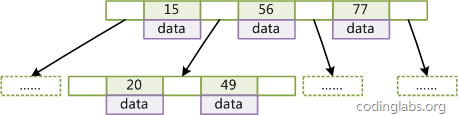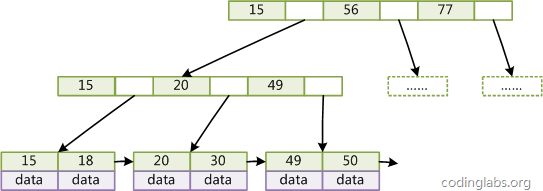# B tree vs B+ tree

- B tree (key+data in every node),  O(log(d)(n))

• d is degree of tree
• h is height of tree, h<= log(d)((n+1)/2)
• non-leaf node has n-1 key and n pointers, d<=n<=2d
• heights of each leaf are same
• nodes are sorted from left to right- B plus tree (key in interior nodes, key+data in leaf nodes),linked leaf nodes for sequential access.

d can be larger than B tree because no data in interior nodes given the same pagesize.

Advantages of B+ trees:

• Because B+ trees don't have data associated with interior nodes, more keys can fit on a page of memory. Therefore, it will require fewer cache misses in order to access data that is on a leaf node.
• The leaf nodes of B+ trees are linked, so doing a full scan of all objects in a tree requires just one linear pass through all the leaf nodes. A B tree, on the other hand, would require a traversal of every level in the tree. This full-tree traversal will likely involve more cache misses than the linear traversal of B+ leaves.

Advantage of B trees:

• Because B trees contain data with each key, frequently accessed nodes can lie closer to the root, and therefore can be accessed more quickly.

;